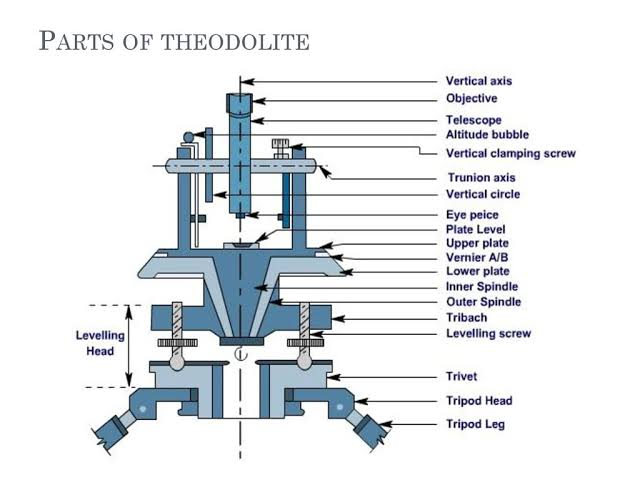Simple Derivation of W=D²L/162, the Formula to Calculate Weight of Steel bars

Diameter and length of steel bars are structural requirements of a building, and they are decided based on design procedures given by IS(Indian Standard) codes. Steel bars are sold in the market in price per weight. So we need to calculate the weight of steel required to estimate cost of construction.
The formula D²L/162 is very common and used frequently to calculate the weight of steel bars. In this post, the simple derivation behind this formula is discussed. I hope you will enjoy it.

The formula to calculate the weight of steel is:

W=D²L/162
Where,
W = Weight of steel in Kg
D = Diameter of steel bars in millimeters (mm)
L= Length of steel bars in meter (m)

Derivation of the Formula:

We know that,
Volume= Cross-sectional area x Length
Weight = Volume × Unit weight
Mathematically,
W = A x L x ρ
A = Area = πD²/4
π (pi) = 3.14
D = Diameter of steel bar
L = Length of steel bar
ρ (Rho) = Density of steel bar = 7850 kg/m³
Therefore,
W = 3.14 x D²/4  x L x 7850 kg
Where D and L are in m

We generally express diameter in millimeter (mm) and length in meter (m) unit. So to make the formula more handy let's take diameter as D mm.
D mm = D/1000 m
Putting D mm value in the expansion of W,
W = 3.141 x (D/1000)²/4 x L x 7850 kg
Or, W = D²L/162 kg
D in mm
& L in m
Share:

Name

Email *

Message *

Featured Post

Theodolite

Theodolite is the most precise instrument used for measuring horizontal and vertical angles. Horizontal and vertical distances can also be...